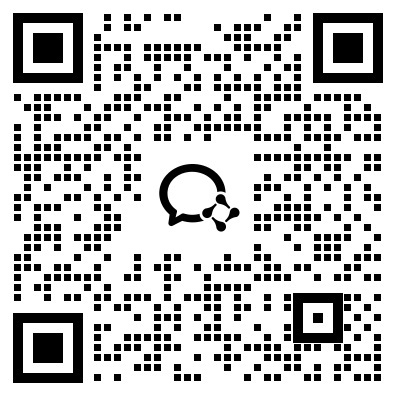﻿ 中山市2019年成人高考高起点数学(理)考试真题及答案_中山成考网

# 中山市2019年成人高考高起点数学(理)考试真题及答案

2019年成人高考高起点数学(理)考试真题及答案

1.设全集U=({,23.4),集合M=(3,4,则CuM =

A.{2,3}B.{2,4]}C(1,4}D.(1,2}

2.函数y = cos4x的最小正周期为

A.I

B，π

D.2π

2、设用: b=0;乙:函数y= kx + b的图像经过坐标原点，则

A.甲是乙的充分条件但不是必要条件

B.用是乙的必要条件但不是充分条件

C.甲是乙的充要条件

D.甲既不是乙的充分条件也不是乙的必要条件

4.已知tana=1/2，则tan(a+π/4)=

A.-3

B.一1/3

c.1/2

D.3

5.函数y=√1-x2“的定义域是

A. {x|x≥-1}

B. {xIx≤1}C. {x|x≤-1}

D. {x|-1≤x≤1} .

6.设0物

D. log;x> 0

7.不等式|x +第>当的解集为A. {x|-1- -1] ，

C. {1>0或x<-1}D. {xkx<0}

8.甲、乙、丙、丁4人排成一行，其中甲、乙必须排在两端，则不同的排法共有

A.3种

B. 8种

C.4种

D.24种

9,若向量a=(1,), b=(1,-1), 则1/2a-3/2b=;

A. (-1,2)

B. (1,-2)

C. (1,2)

D. (-1,-2)

11,y=x2- 4x- 的图像与x轴交于A.B两点，则丨AB 丨=

A.3

B 4

C.5

D.6

14.若直线mx +y-1= 0与直线4x+ 2y+1= 0平行，则m=

A. -1

B.0

C.1

D.2

15.在等比数列中，若a4a5= 6，则a2a3a6a7,=

A.36

B.24

C. 12

D.6

16.已知函数f(x)的定义域为R，且f(2x)=4x+ 1,则f(1) =

A.5

B.3

C.7

17.甲、乙各独立地射击一次，己知甲射中10环的概率为0.9,乙射中10换的概率为0.5，则甲、乙都射中10环的概率为

A.0.45

B.0.25

C.0.2

D.0,75

22.缺少题干，仅供参考

S2o= 20a1 +- d=-55

23.AB= V6

24.缺少题干，仅供参考﻿

## 中山成考网在线客服